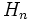# IAPS of groups

## Contents

BEWARE! This term is nonstandard and is being used locally within the wiki. [SHOW MORE]

This article gives a basic definition in the following area: APS theory
View other basic definitions in APS theory |View terms related to APS theory |View facts related to APS theory
This article defines the notion of group object in the category of IAPSs|View other types of group objects

## Definition

An IAPS of groups is an IAPS over the category of groups. More specifically an IAPS$(G,\Phi)$ is the following data:

• For each natural number$n$, a group denoted$G_n$
• For each ordered pair$(m,n)$ of natural numbers, an injective homomorphism$\Phi_{m,n}:G_m \times G_n \to G_{m+n}$

Satisfying the following compatibility conditions:

For$g, h, k$ in$G_m, G_n, G_p$ respectively:$\Phi_{m+n,p} (\Phi_{m,n}(g,h),k) = \Phi_{m,n+p} (g, \Phi_{n,p}(h,k))$.

The above condition is termed an associativity condition.

We may assume$G_0$ as the trivial group and define$\Phi_{m,0}$ and$\Phi_{0,n}$ as trivial paddings.

Note that if we remove the condition of injectivity, we get an APS of groups.

## Constructions

### Sub-IAPS

Further information: sub-IAPS of groups

Let$(G,\Phi)$ be an IAPS of groups. A sub-IAPS$H$ associated to every$n$ a subgroup$H_n$ of$G_n$ such that the image of$H_m \times H_n$ under$\Phi_{m,n}$ lies inside$H_{m+n}$. Note that this is the same as a sub-APS of groups because the injectivity condition comes for free.